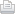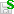https://doi.org/10.5194/gmd-2020-146
https://doi.org/10.5194/gmd-2020-146
Status: this preprint was under review for the journal GMD but the revision was not accepted.

# Quasi-hydrostatic equations for climate models and the study on linear instability

Robert Nigmatulin and Xiulin Xu

Abstract. An advanced “quasi-hydrostatic approximation” of 3-dimensional atmospheric-dynamics equations is proposed and justified with the practical goal to optimize atmospheric modelling at scales ranging from meso meteorology to global climate. For the vertically quasi-hydrostatic flow with inertial forces negligibly small compared to gravity forces, the asymptotically exact equation for vertical velocity is obtained. In the closed system of hydro/thermodynamic equations, the pressure is determined by the total air mass above, so that mass instead of pressure is considered as a dependent variable. In such a system, the sound waves are filtered, though the horizontal inertia forces are taken into account in the horizontal momentum conservation equations. The major practical result is an asymptotically exact equation for vertical velocity in the quasi-hydrostatic system of the atmospheric dynamics equations.

Investigation of the stability of solutions to the system in response to small shortwave perturbations has shown that solutions have the property of shortwave instability. There are situations when the growth rate of the perturbation amplitude tends to infinity. It means that the Cauchy problem for such equations may be ill-posed. Its formulation can become conditionally correct if solutions are sought in a limited class of sufficiently smooth functions whose Fourier harmonics tend to zero reasonably quickly when the wavelengths of the perturbations approach zero. Thus, the numerical scheme for the quasi-hydrostatic equations using the finite-difference method requires an adequately selected pseudo-viscosity to eliminate the instability caused by perturbations with wavelengths of the order of the grid size. The result is useful for choosing appropriate vertical and horizontal grid sizes for modelling to avoid shortwave instability associated with the property of the system of equations. Implementation of pseud-viscosities helps to smoothen or suppress the perturbations that occur during modelling.

Share

Robert Nigmatulin and Xiulin Xu

Status: closed
Status: closed
AC: Author comment | RC: Referee comment | SC: Short comment | EC: Editor comment- Printer-friendly version- Supplement

Status: closed
Status: closed
AC: Author comment | RC: Referee comment | SC: Short comment | EC: Editor comment- Printer-friendly version- Supplement

Robert Nigmatulin and Xiulin Xu

Robert Nigmatulin and Xiulin Xu

## Viewed

Total article views: 1,156 (including HTML, PDF, and XML)
HTML PDF XML Total BibTeX EndNote
895 229 32 1,156 20 23
• HTML: 895
• PDF: 229
• XML: 32
• Total: 1,156
• BibTeX: 20
• EndNote: 23